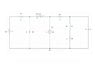# Critically damped, or underdamped, or overdamped?

wiz0r

## Homework Statement

Hello, I got to solve the following second order transient circuit. Obviously, what I need to do first is to find if its critically damped, underdamped, or overdamped.

The circuit can be found in the attachment.

## Homework Equations

Depends, weather the circuit is critically damped, underdamped, or overdamped.

## The Attempt at a Solution

So, what I do is to remove the voltage source by a short and the current source by an open circuit, then assume the switch will be opened at t>0, so there will be an open circuit where the switch was. Then try to find if its a series RLC or a parallel RLC, exactly, how can I do that? I need to know if its a series RLC or parallel RLC to determine weather the circuit is critically damped, underdamped, or overdamped.

Any help would be gladly appreciated!

#### Attachments

•fullcircuit.JPG
22.9 KB · Views: 777

Homework Helper
You don't start off by assuming one of the three possible damping modes. Just find the voltage across the capacitor and current through the inductor at steady state before the voltage and current source and shorted and removed respectively and the switch is opened at t=0. Once you do that you can write out a couple of mesh analysis equations and solve them for current through inductor and capacitor for t>0. Your initial conditions to solve the DE would be the current through the inductor and voltage across the capacitor at t=0.

Only after you have done all these, then you'll be able to tell if this is either under damped, critically damped or overdamped from the mathematical expresssion.

misho
I'd do the following:

1. get the initial voltage one either side of the inductor (they are the same 4 V).
2. after the switch closes, get a Norton or Thevenin equivalent for the entire part to the left of the inductor.
3. make a supernode around the inductor. write an equation for it.
4. use that V = LdI/dt for an inductor.
5. solve the system you get.

If all you need is the voltage across the capacitor or something at the end like that, it might be worth it to treat this problem as cascaded 2-port networks and find the T matrix for each.

EDIT: whether or not your circuit is over-/under-/critically damped just depends on the solution to the equation you get. There's no point in trying to guess what it is beforehand.

wiz0r
Oh, I get it now. Thanks for the answer, guys.

However, I had something wrong. The switch is actually closed, and it will open at t = 0. Also, I need to find V(t) of resistance number 4 (R4) Could any of you tell me what would you do? Basically, a hint to start doing the problem?

Thanks a lot.

misho
1. use KCL to get initial current through L and voltage across C (maybe find an equivalent cct for the left part, although, I doubt it would be any easier to do that)
2. make supernode around inductor
3. use V=L dI/dt for inductor
4. solve the differential equations
5. the voltage across R4 is R4 * I (where I goes through R4)